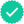Question

n a bag of M & M’s there are 80 M & Ms, with 11 red ones, 12 orange ones, 20 blue ones, 11 green ones, 18 yellow ones, and 8 brown ones. They are mixed up so that each candy piece is equally likely to be selected if we pick one.a.If we select one at random, what is the probability that it is red?b.If we select one at random, what is the probability that it is not blue?c.If we select one at random, what is the probability that it is red or orange?d.If we select one at random, then put it back, mix them well and select another one, what is the probability that both the first and second ones are blue?e.If we select one, keep it, and then select a second one, what is the probability that the first one is red and the second one is green?

Expert SolutionKnowledge Booster••